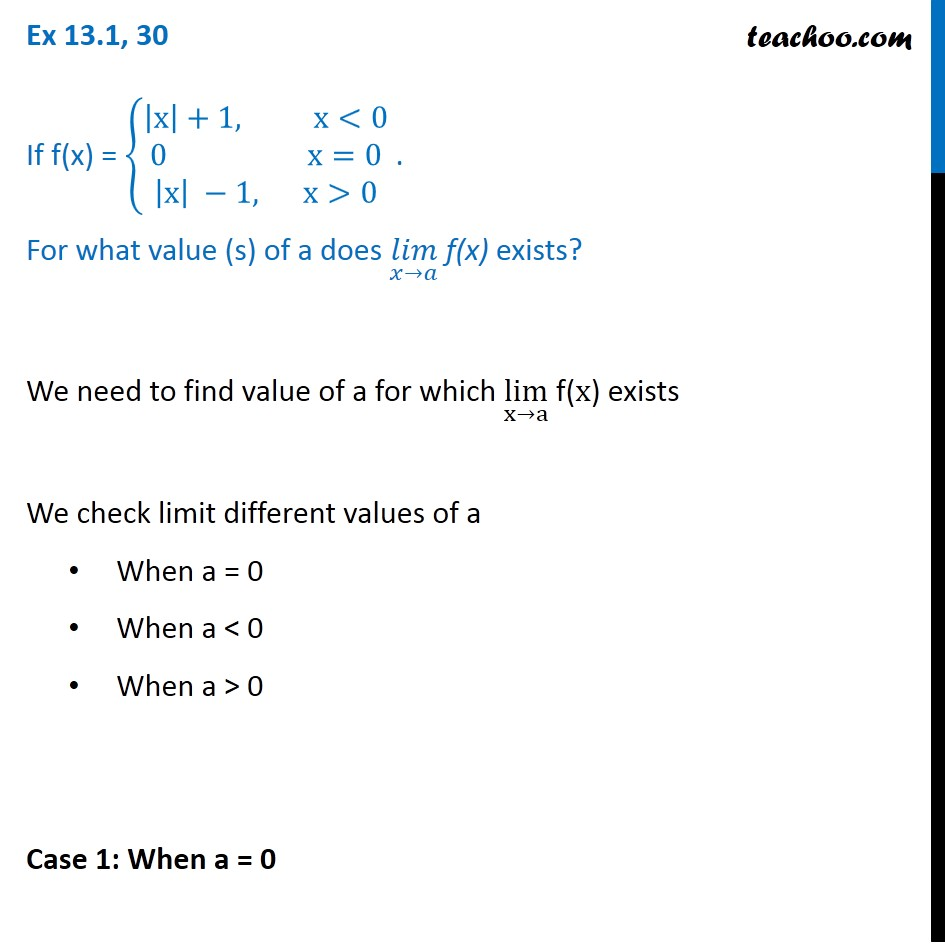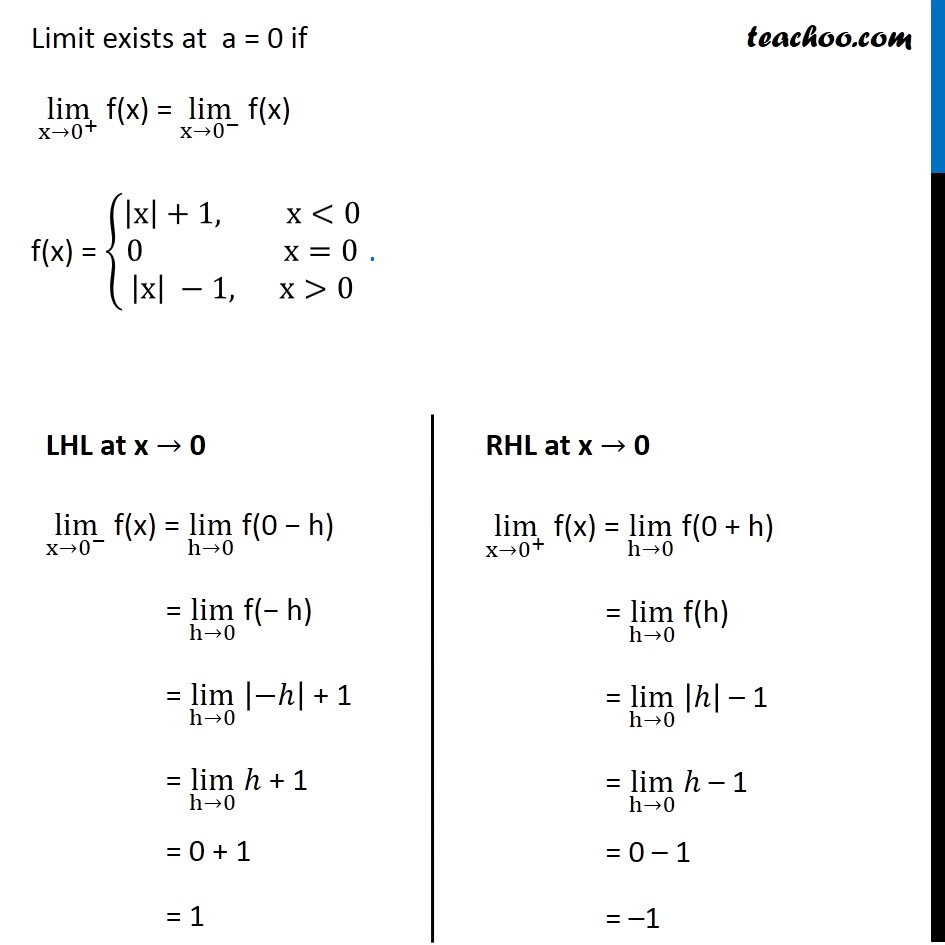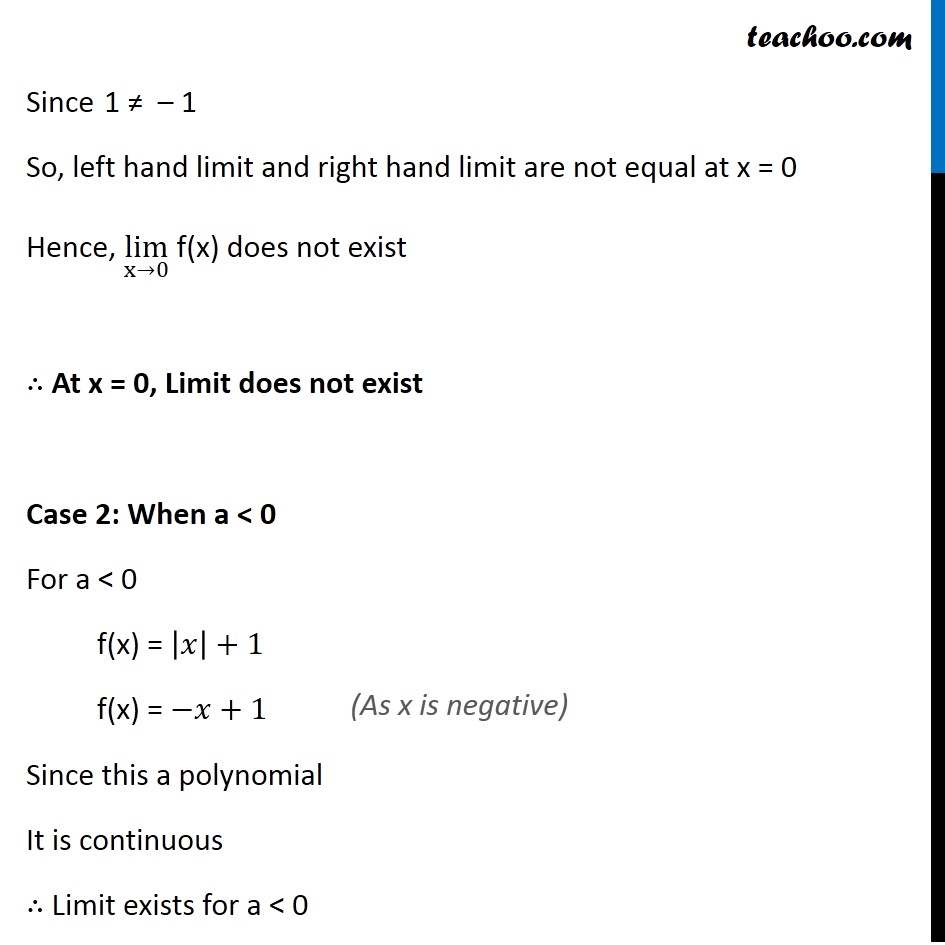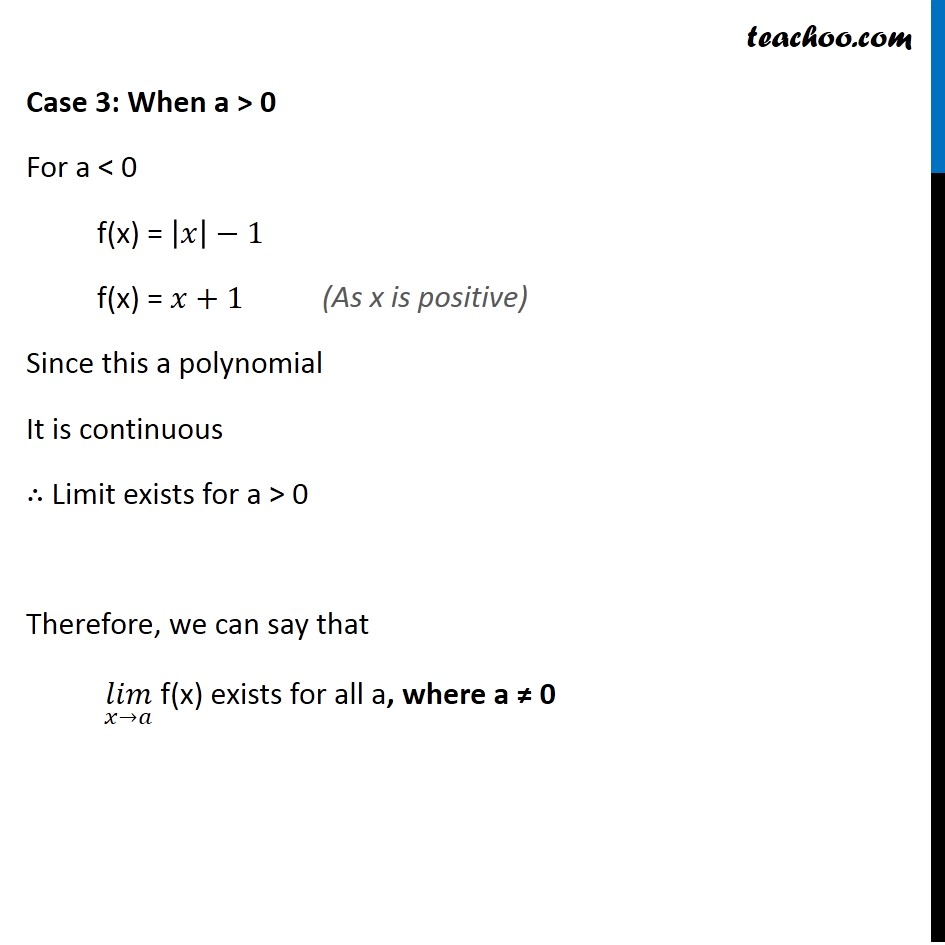1. Chapter 13 Class 11 Limits and Derivatives (Term 1 and Term 2)
2. Concept wise
3. Limits - Limit exists

Transcript

Ex 13.1, 30 If f(x) = {█(|x|+1, x<0@0 x=0@|x| −1, x>0)┤ . For what value (s) of a does (𝑙𝑖𝑚)┬(𝑥→𝑎) f(x) exists? We need to find value of a for which lim┬(x→a) f(x) exists We check limit different values of a When a = 0 When a < 0 When a > 0 Case 1: When a = 0 Limit exists at a = 0 if lim┬(x→0^+ ) " f(x) = " lim┬(x→0^− ) " f(x)" f(x) = {█(|x|+1, x<0@0 x=0@|x| −1, x>0)┤ . LHL at x → 0 lim┬(x→0^− ) f(x) = lim┬(h→0) f(0 − h) = lim┬(h→0) f(− h) = lim┬(h→0) |−ℎ| + 1 = lim┬(h→0) ℎ + 1 = 0 + 1 = 1 RHL at x → 0 lim┬(x→0^+ ) f(x) = lim┬(h→0) f(0 + h) = lim┬(h→0) f(h) = lim┬(h→0) |ℎ| – 1 = lim┬(h→0) ℎ – 1 = 0 – 1 = –1 Since 1 ≠ – 1 So, left hand limit and right hand limit are not equal at x = 0 Hence, lim┬(x→0) f(x) does not exist ∴ At x = 0, Limit does not exist Case 2: When a < 0 For a < 0 f(x) = |𝑥|+1 f(x) = −𝑥+1 Since this a polynomial It is continuous ∴ Limit exists for a < 0 (As x is negative) Case 3: When a > 0 For a < 0 f(x) = |𝑥|−1 f(x) = 𝑥+1 Since this a polynomial It is continuous ∴ Limit exists for a > 0 Therefore, we can say that (𝑙𝑖𝑚)┬(𝑥→𝑎) f(x) exists for all a, where a ≠ 0 (As x is positive)